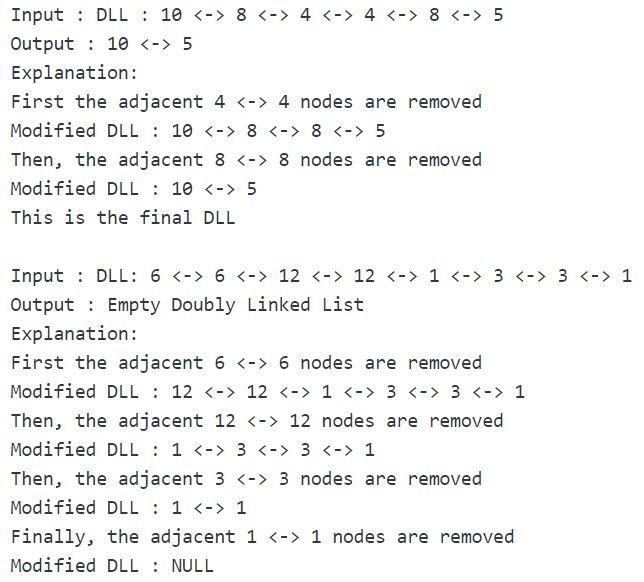Related Articles
• Last Updated : 29 Jun, 2020

Given a doubly linked list. The problem is to remove all adjacent duplicate nodes from the list such that the final modified doubly linked list does not contain any adjacent duplicate nodes.

Examples:## Recommended: Please try your approach on {IDE} first, before moving on to the solution.

Approach: The approach uses stack to keep track of the adjacent nodes at any point in the modified Doubly Linked List.

Algorithm:

```delAdjacentDuplicates(head_ref)
Create an empty stack st
Declare current, next, top
while current != NULL
if isEmpty(st) or current->data != peek(st)->data
push current on to the stack st
current = current->next
else
next = current->next
top = peek(st)
pop element from st
delete node 'current'
delete node 'top'
current = next
```

peek(st) operation returns the value at the top of the stack. The algorithm to delete a node n from the doubly linked list using pointer to the node n is discussed in this post.

 `/* C++ implementation to delete adjacent duplicate nodes  ` `   ``from the Doubly Linked List */` `#include ` ` `  `using` `namespace` `std; ` ` `  `/* a node of the doubly linked list */` `struct` `Node { ` `    ``int` `data; ` `    ``struct` `Node* next; ` `    ``struct` `Node* prev; ` `}; ` ` `  `/* Function to delete a node in a Doubly Linked List. ` `   ``head_ref --> pointer to head node pointer. ` `   ``del  -->  pointer to node to be deleted. */` `void` `deleteNode(``struct` `Node** head_ref, ``struct` `Node* del) ` `{ ` `    ``/* base case */` `    ``if` `(*head_ref == NULL || del == NULL) ` `        ``return``; ` ` `  `    ``/* If node to be deleted is head node */` `    ``if` `(*head_ref == del) ` `        ``*head_ref = del->next; ` ` `  `    ``/* Change next only if node to be deleted is NOT the last node */` `    ``if` `(del->next != NULL) ` `        ``del->next->prev = del->prev; ` ` `  `    ``/* Change prev only if node to be deleted is NOT the first node */` `    ``if` `(del->prev != NULL) ` `        ``del->prev->next = del->next; ` ` `  `    ``/* Finally, free the memory occupied by del*/` `    ``free``(del); ` `} ` ` `  `/* function to delete adjacent duplicate nodes from ` `   ``the Doubly Linked List */` `void` `delAdjacentDupNodes(``struct` `Node** head_ref) ` `{ ` `    ``// an empty stack 'st' ` `    ``stack st; ` ` `  `    ``struct` `Node* current = *head_ref; ` ` `  `    ``/* traverse the doubly linked list */` `    ``while` `(current != NULL) { ` `        ``/* if stack 'st' is empty or if current->data != st.top()->data ` `           ``push 'current' on to the stack 'st' */` `        ``if` `(st.empty() || current->data != st.top()->data) { ` `            ``st.push(current); ` ` `  `            ``/* move to the next node */` `            ``current = current->next; ` `        ``} ` ` `  `        ``// else current->data == st.top()->data ` `        ``else` `{ ` `            ``/* pointer to the node next to the 'current' node */` `            ``struct` `Node* next = current->next; ` ` `  `            ``/* pointer to the node at the top of 'st' */` `            ``struct` `Node* top = st.top(); ` ` `  `            ``/* remove top element from 'st' */` `            ``st.pop(); ` ` `  `            ``/* delete 'current' node from the list */` `            ``deleteNode(head_ref, current); ` ` `  `            ``/* delete 'top' node from the list */` `            ``deleteNode(head_ref, top); ` ` `  `            ``/* update 'current' */` `            ``current = next; ` `        ``} ` `    ``} ` `} ` ` `  `/* Function to insert a node at the beginning  ` `   ``of the Doubly Linked List */` `void` `push(``struct` `Node** head_ref, ``int` `new_data) ` `{ ` `    ``/* allocate node */` `    ``struct` `Node* new_node = (``struct` `Node*)``malloc``(``sizeof``(``struct` `Node)); ` ` `  `    ``/* put in the data  */` `    ``new_node->data = new_data; ` ` `  `    ``/* since we are adding at the beginning, ` `    ``prev is always NULL */` `    ``new_node->prev = NULL; ` ` `  `    ``/* link the old list off the new node */` `    ``new_node->next = (*head_ref); ` ` `  `    ``/* change prev of head node to new node */` `    ``if` `((*head_ref) != NULL) ` `        ``(*head_ref)->prev = new_node; ` ` `  `    ``/* move the head to point to the new node */` `    ``(*head_ref) = new_node; ` `} ` ` `  `/* Function to print nodes in a given doubly linked list */` `void` `printList(``struct` `Node* head) ` `{ ` `    ``if` `(head == NULL) ` `        ``cout << ``"Empty Doubly Linked List"``; ` ` `  `    ``while` `(head != NULL) { ` `        ``cout << head->data << ``" "``; ` `        ``head = head->next; ` `    ``} ` `} ` ` `  `/* Driver program to test above functions*/` `int` `main() ` `{ ` `    ``/* Start with the empty list */` `    ``struct` `Node* head = NULL; ` ` `  `    ``/* Create the doubly linked list 10<->8<->4<->4<->8<->5 */` `    ``push(&head, 5); ` `    ``push(&head, 8); ` `    ``push(&head, 4); ` `    ``push(&head, 4); ` `    ``push(&head, 8); ` `    ``push(&head, 10); ` ` `  `    ``cout << ``"Doubly linked list before deletion:n"``; ` `    ``printList(head); ` ` `  `    ``/* delete adjacent duplicate nodes */` `    ``delAdjacentDupNodes(&head); ` ` `  `    ``cout << ``"nDoubly linked list after deletion:n"``; ` `    ``printList(head); ` ` `  `    ``return` `0; ` `} `

Output:

```Doubly linked list before deletion:
10 8 4 4 8 5
10 5
```

Time Complexity: O(n)
Auxiliary Space: O(n), in worst case when there are no adjacent duplicate nodes.

This article is contributed by Ayush Jauhari. If you like GeeksforGeeks and would like to contribute, you can also write an article using contribute.geeksforgeeks.org or mail your article to contribute@geeksforgeeks.org. See your article appearing on the GeeksforGeeks main page and help other Geeks.